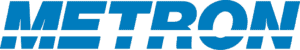## Bellman’s Equation and Stochastic Dynamic Programming

Stochastic dynamic programming is the use of linear, non-linear, and mixed-integer programming integrated with probability, statistics, and variational and functional analysis to provide answers to optimization problems in unstable spaces. It provides a robust mathematical methodology for deriving optimal solutions to sequential decision problems – problems in which we begin in one state, make a decision that randomly affects our next state, and repeat this process.

Let’s imagine we want to determine the best route to take for our morning commute. To do so, we must first decide what we mean by “best.” Is our objective to optimize our trip based on travel time, mileage traveled, arriving at a certain time? After we choose this objective, we can determine the cost function of a particular decision by examining its impact. How does taking a left at Albuquerque affect our current position, travel time, and remaining distance to the destination? These state variables affect feasible future decisions and outcomes. We change state variables by adjusting control variables at any given stage. For example, we can choose to turn left or right at an intersection, or we can chose to exit the highway or continue down the road. Each choice we make determines the state variable values we have at the next stage. Ideally we could find a policy rule that tells us which control variable values to choose at each possible state value to reach the optimal outcome: if I am at this intersection at this time, what is my best option to get to work?

Bellman discovered that one way to find that policy rule is through “backward induction,” measuring a decision’s value based on how it changes the possible final outcome we can reach. We then move to the previous stage and determine the cost value for those decisions. This recursive process is the foundation of what is known as stochastic dynamic programming.

Stochastic dynamic programming problems can be solved completely for relatively small-state problems using various established techniques including value iteration and policy iteration. For large-state problems, methods exist under the umbrella of approximate dynamic programming such as rollout, in which the Bellman recursion is evaluated for a manageable number of steps and an assumed near-optimal base policy (based on separability or other considerations) is used to approximate optimality thereafter.

## Making Decisions in Variable Timeframe and Uncertain Environment​

For real-world problems, the strategy we choose may change based on the length of time we are considering. For example, we might apply a different strategy for short-term stock market investments than we would in the longer-term or when a major fluctuation affects our investment. We may also face uncertainty about state variable changes from control variables or outside influences. Markov decision processes, in which incremental rewards are accumulated by sequentially applying possible control actions at appropriate decision steps, and for which available control choices at each step have estimable random consequences, gives us a framework of choosing the optimal decision over the short term while making data-driven estimations of variables.​